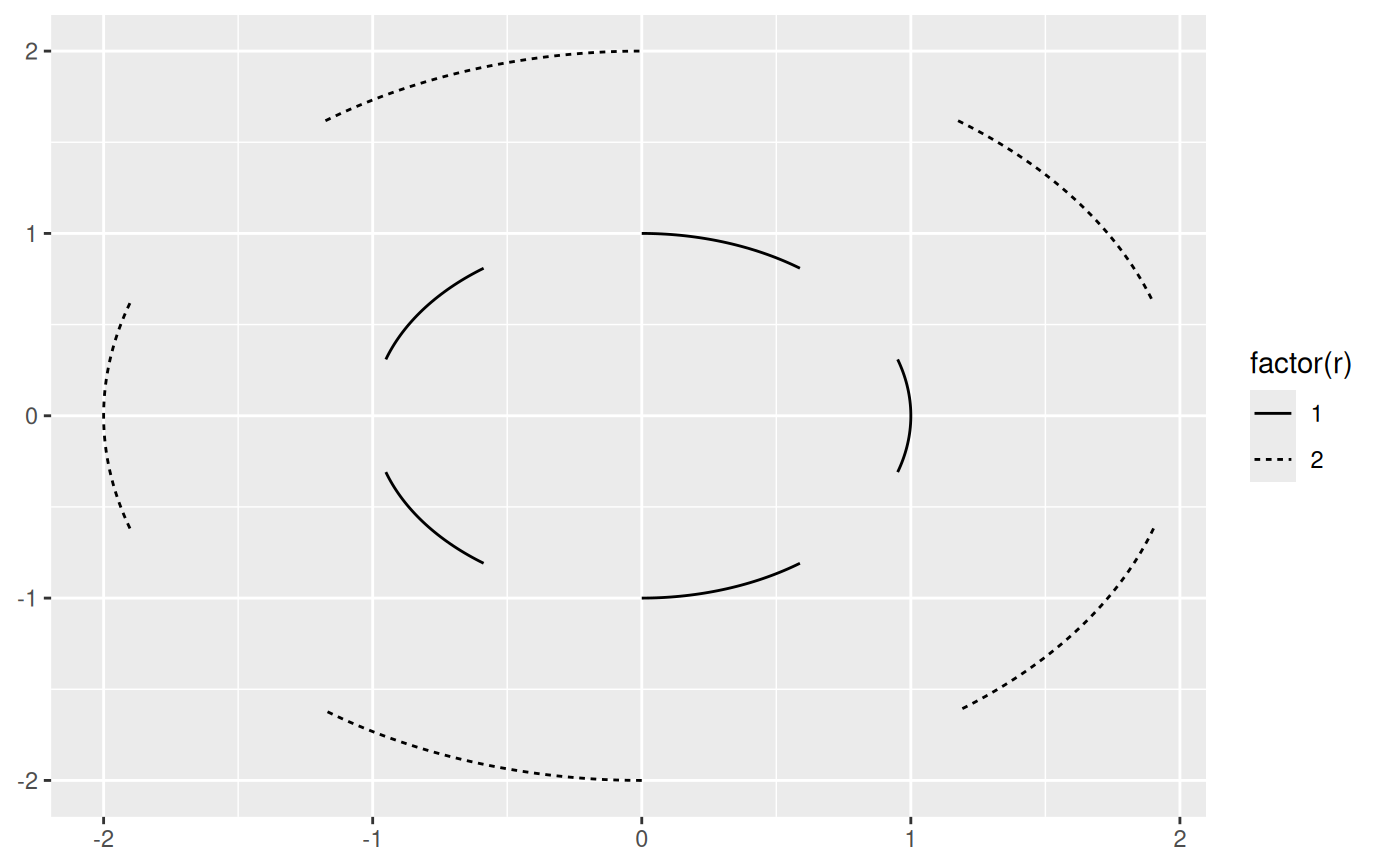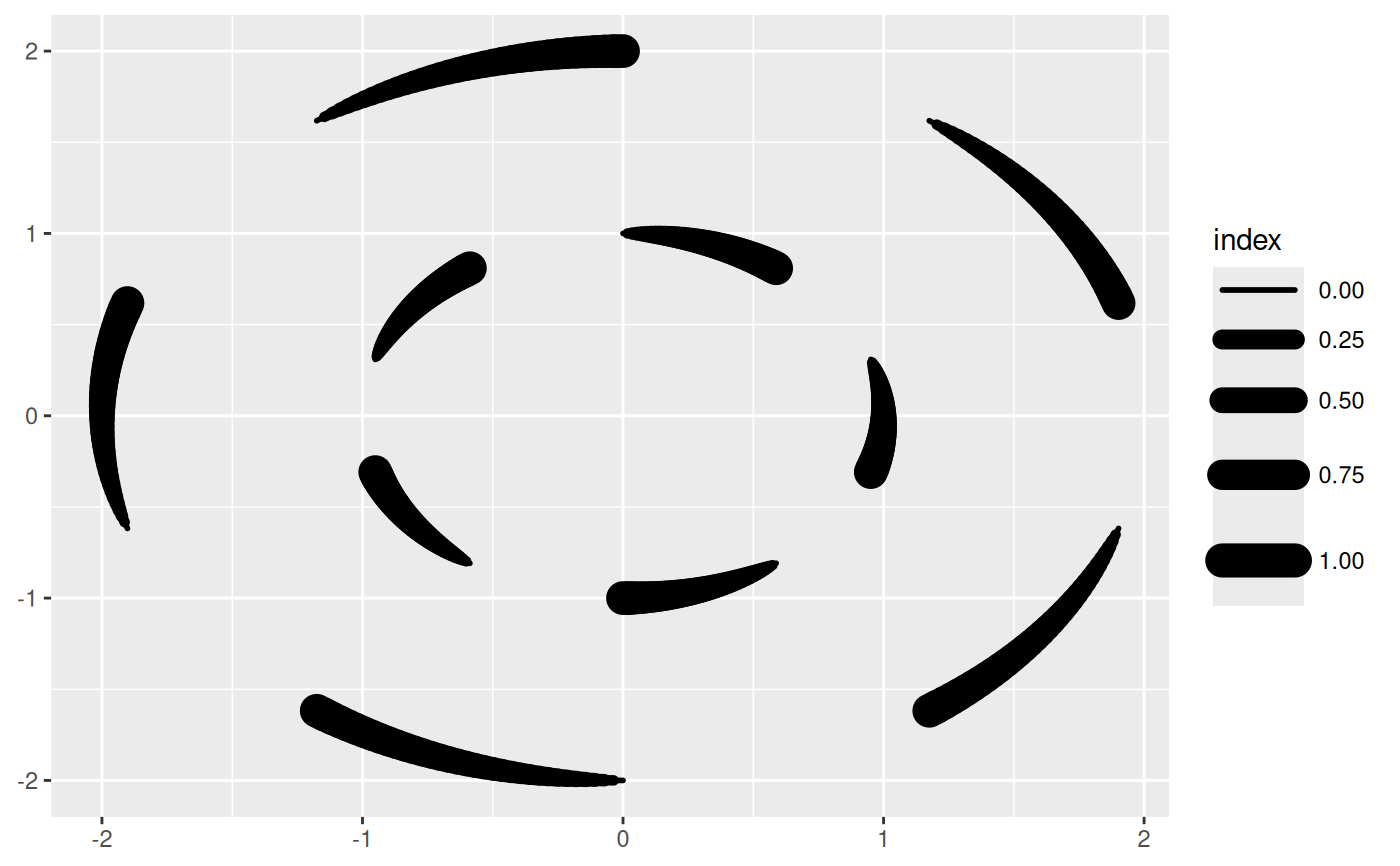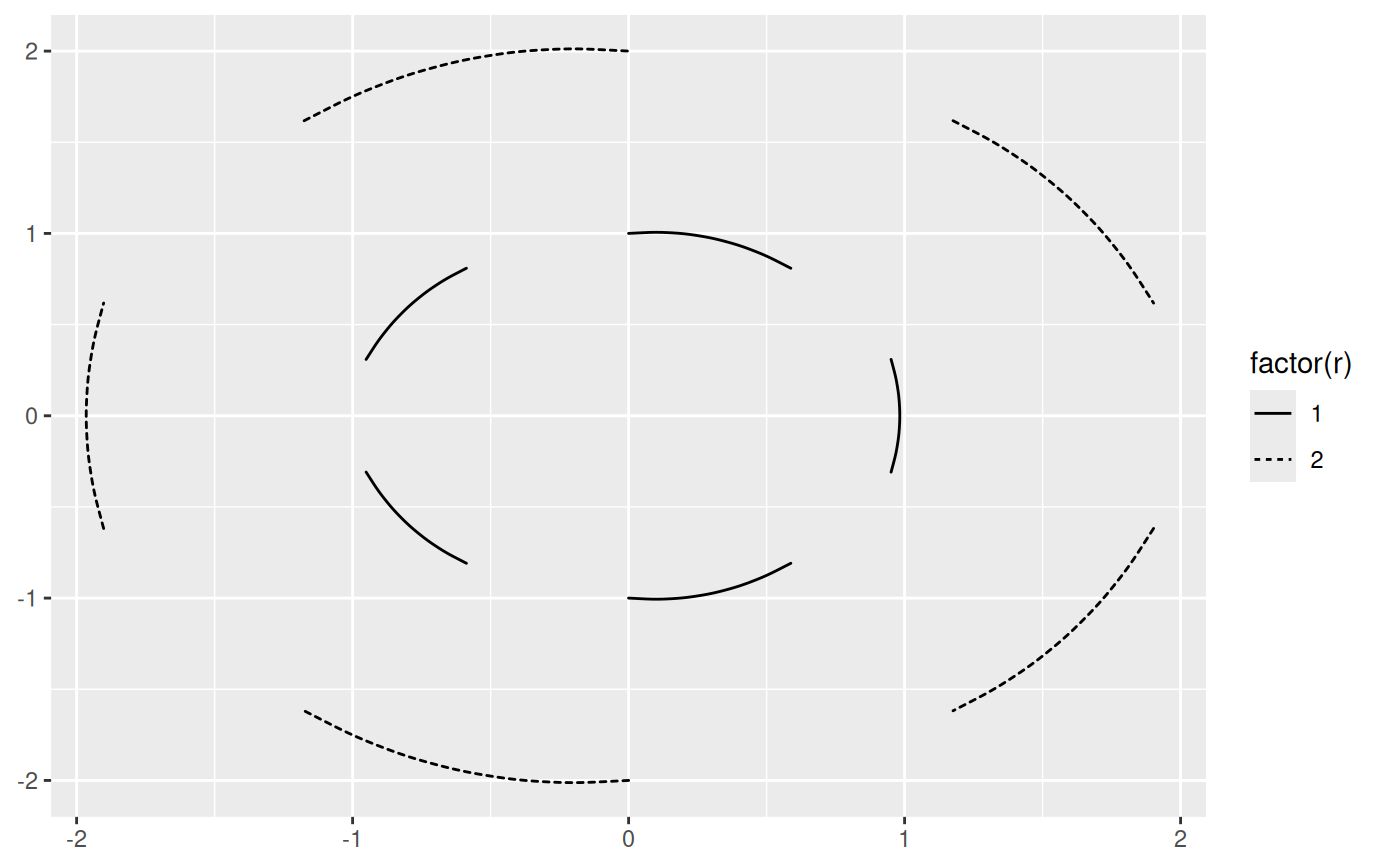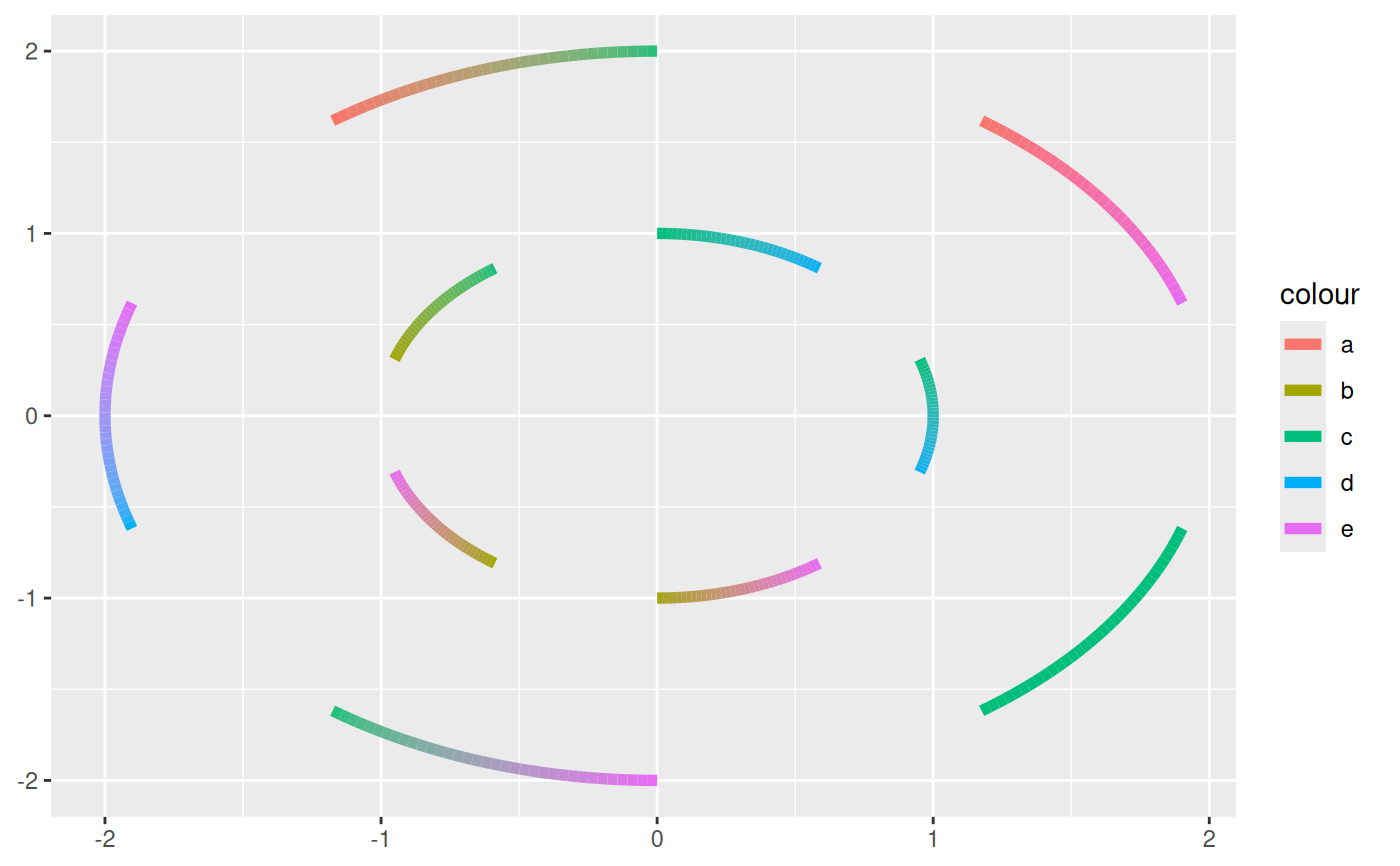This set of stats and geoms makes it possible to draw circle segments based on a center point, a radius and a start and end angle (in radians). These functions are intended for cartesian coordinate systems and makes it possible to create circular plot types without using the ggplot2::coord_polar() coordinate system.

stat_arc(
mapping = NULL,
data = NULL,
geom = "arc",
position = "identity",
na.rm = FALSE,
show.legend = NA,
n = 360,
inherit.aes = TRUE,
...
)

geom_arc(
mapping = NULL,
data = NULL,
stat = "arc",
position = "identity",
n = 360,
arrow = NULL,
lineend = "butt",
na.rm = FALSE,
show.legend = NA,
inherit.aes = TRUE,
...
)

stat_arc2(
mapping = NULL,
data = NULL,
geom = "path_interpolate",
position = "identity",
na.rm = FALSE,
show.legend = NA,
n = 360,
inherit.aes = TRUE,
...
)

geom_arc2(
mapping = NULL,
data = NULL,
stat = "arc2",
position = "identity",
n = 360,
arrow = NULL,
lineend = "butt",
na.rm = FALSE,
show.legend = NA,
inherit.aes = TRUE,
...
)

stat_arc0(
mapping = NULL,
data = NULL,
geom = "arc0",
position = "identity",
na.rm = FALSE,
show.legend = NA,
inherit.aes = TRUE,
...
)

geom_arc0(
mapping = NULL,
data = NULL,
stat = "arc0",
position = "identity",
ncp = 5,
arrow = NULL,
lineend = "butt",
na.rm = FALSE,
show.legend = NA,
inherit.aes = TRUE,
...
)

## Arguments

mapping

Set of aesthetic mappings created by aes(). If specified and inherit.aes = TRUE (the default), it is combined with the default mapping at the top level of the plot. You must supply mapping if there is no plot mapping.

data

The data to be displayed in this layer. There are three options:

If NULL, the default, the data is inherited from the plot data as specified in the call to ggplot().

A data.frame, or other object, will override the plot data. All objects will be fortified to produce a data frame. See fortify() for which variables will be created.

A function will be called with a single argument, the plot data. The return value must be a data.frame, and will be used as the layer data. A function can be created from a formula (e.g. ~ head(.x, 10)).

geom

The geometric object to use to display the data, either as a ggproto Geom subclass or as a string naming the geom stripped of the geom_ prefix (e.g. "point" rather than "geom_point")

position

Position adjustment, either as a string naming the adjustment (e.g. "jitter" to use position_jitter), or the result of a call to a position adjustment function. Use the latter if you need to change the settings of the adjustment.

na.rm

If FALSE, the default, missing values are removed with a warning. If TRUE, missing values are silently removed.

show.legend

logical. Should this layer be included in the legends? NA, the default, includes if any aesthetics are mapped. FALSE never includes, and TRUE always includes. It can also be a named logical vector to finely select the aesthetics to display.

n

the smoothness of the arc. Sets the number of points to use if the arc would cover a full circle

inherit.aes

If FALSE, overrides the default aesthetics, rather than combining with them. This is most useful for helper functions that define both data and aesthetics and shouldn't inherit behaviour from the default plot specification, e.g. borders().

...

Other arguments passed on to layer(). These are often aesthetics, used to set an aesthetic to a fixed value, like colour = "red" or size = 3. They may also be parameters to the paired geom/stat.

stat

The statistical transformation to use on the data for this layer, either as a ggproto Geom subclass or as a string naming the stat stripped of the stat_ prefix (e.g. "count" rather than "stat_count")

arrow

Arrow specification, as created by grid::arrow().

lineend

Line end style (round, butt, square).

ncp

the number of control points used to draw the arc with curveGrob. Determines how well the arc approximates a circle section

## Details

An arc is a segment of a line describing a circle. It is the fundamental visual element in donut charts where the length of the segment (and conversely the angular span of the segment) describes the proportion of an entety.

## Aesthetics

geom_arc understand the following aesthetics (required aesthetics are in bold):

• x0

• y0

• r

• start

• end

• color

• linewidth

• linetype

• alpha

• lineend

## Computed variables

x, y

The start coordinates for the segment

xend, yend

The end coordinates for the segment

curvature

The curvature of the curveGrob to match a circle

geom_arc_bar() for drawing arcs with fill

## Examples

# Lets make some data
arcs <- data.frame(
start = seq(0, 2 * pi, length.out = 11)[-11],
end = seq(0, 2 * pi, length.out = 11)[-1],
r = rep(1:2, 5)
)

# Behold the arcs
ggplot(arcs) +
geom_arc(aes(x0 = 0, y0 = 0, r = r, start = start, end = end,
linetype = factor(r)))# Use the calculated index to map values to position on the arc
ggplot(arcs) +
geom_arc(aes(x0 = 0, y0 = 0, r = r, start = start, end = end,
size = after_stat(index)), lineend = 'round')# The 0 version maps directly to curveGrob instead of calculating the points
# itself
ggplot(arcs) +
geom_arc0(aes(x0 = 0, y0 = 0, r = r, start = start, end = end,
linetype = factor(r)))# The 2 version allows interpolation of aesthetics between the start and end
# points
arcs2 <- data.frame(
angle = c(arcs$start, arcs$end),
r = rep(arcs\$r, 2),
group = rep(1:10, 2),
colour = sample(letters[1:5], 20, TRUE)
)

ggplot(arcs2) +
geom_arc2(aes(x0 = 0, y0 = 0, r = r, end = angle, group = group,
colour = colour), size = 2)